# CHEMICAL EQUILIBRIUM: INTRODUCTION

Chemical equilibrium deals with to what extent a chemical reaction proceeds.

It is observed that, in most of the chemical reactions, the reactants are not completely converted to products. The reaction proceeds to certain extent and reaches a state at which the concentrations of both reactants and products remain constant with time. This state is generally referred to as equilibrium state

In these reactions, not only the conversion of reactants to products occurs, but also the conversion of products to reactants is possible. These reactions are known as reversible reactions. They reach equilibrium state where the number of reactant species converted to products becomes equal to the number of product species converted to reactants at a given instant of time i.e., the rate of forward reaction becomes equal to the rate of backward reaction. That is why at equilibrium, there is no observable change in the concentration of reactants and products. The reaction is said to be halted and no further conversion of reactants is possible under given set of conditions.

Chemical equilibrium deals with these reversible reactions, which reach equilibrium state. The scope of chemical equilibrium includes the study of  characteristics and factors affecting the chemical equilibria.

## IRREVERSIBLE AND REVERSIBLE REACTIONS

Irreversible reaction: A reaction that occur only in one direction is called an irreversible reaction i.e., only the reactants are converted to products and the conversion of products to reactants is not possible.

The single headed arrow (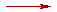) is used to indicate the irreversible reactions.

E.g.

1) The combustion of methane is an irreversible reaction since it is not possible to convert the products (carbon dioxide and water) back to the reactants (methane and oxygen).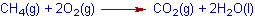2) The decomposition of potassium chlorate is also an irreversible reaction. It is not possible to prepare potassium chlorate directly from KCl and O2.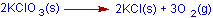Reversible reaction : A reaction that occurs in both forward and backward directions is called reversible reaction. In a reversible reaction, the reactants are converted into products and the products can also be converted back to the reactants.

The half headed arrows (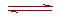) are used to indicate the reversible reactions.

E.g. The following reactions are reversible reactions since they occur in both directions.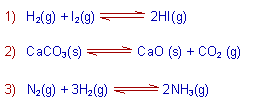The chemical equilibrium is possible in reversible reactions only.

## CHEMICAL EQUILIBRIUM: DEFINITION

Chemical equilibrium: The state at which the rate of forward reaction becomes equal to the rate of backward reaction is called chemical equilibrium.

Explanation: Initially the rate of forward reaction is greater than the rate of backward reaction. However during the course of reaction, the concentration of reactants decreases and the concentration of products increases. Since the rate of a reaction is directly proportional to the concentration, the rate of forward reaction decreases with time, whereas the rate of backward reaction increases.

At certain stage, both the rates become equal. From this point onwards, there will be no change in the concentrations of both reactants and products with time. This state is called as equilibrium state.

The state of chemical equilibrium can be shown graphically as follows:## CHARACTERISTICS OF CHEMICAL EQUILIBRIUM

1) At equilibrium state, the rates of forward and backward reactions are equal.

2) The observable properties such as pressure, concentration, color, density, viscosity etc., of the system remain unchanged with time.

3) The chemical equilibrium is a dynamic equilibrium, because both the forward and backward reactions continue to occur even though it appears static externally.

The concentrations of reactants and products do not change with time but their inter conversion continue to occur.

4) The chemical equilibrium can be reached by starting the reaction either from the reactants side or from the products side.

5) Both pressure and concentration affect the state of equilibrium but do not affect the equilibrium constant.

6) However, temperature can affect both the state of equilibrium as well as the equilibrium constant.

6) A positive catalyst can increase the rates of both forward and backward reactions and thus helping the system to attain the equilibrium faster. But it does not affect the state of equilibrium and the equilibrium constant.

## TYPES OF CHEMICAL EQUILIBRIA

The chemical equilibria are classified into two types: 1) Homogeneous equilibrium and 2) Heterogeneous equilibrium.

1) Homogeneous equilibrium: A chemical equilibrium is said to be homogeneous if all the substances (reactants and products) at equilibrium are in the same phase.

E.g.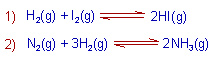2) Heterogeneous equilibrium: A chemical equilibrium is said to be heterogeneous if all the substances at equilibrium are not in the same phase.

E.g.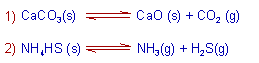## LAW OF MASS ACTION

The law of mass action was proposed by Guld berg and Wage. It can be stated as:

The rate of a reaction at an instant of time is proportional to the product of active masses of the reactants at that instant of time under given conditions.

The active masses for different substances and systems can be expressed as mentioned below.

i) For dilute solutions, the molar concentrations are taken as active masses.

ii) For gases at low pressures, the partial pressures are taken as active masses. However the molar concentrations can also be taken as active masses.

iii) The active masses of pure solids and pure liquids are taken as unity since their active masses (or concentrations) are independent of their quantities taken.

E.g. For the reaction:

N2(g) + 3H2(g)2NH3(g)

The rate of the reaction at an instant of time can be expressed as:

r ∝ [N2] [H2]3

or

r ∝ pN2.pH23

Where:

[N2] and [H2] are the molar concentrations of gases.

pN2 and pH2 are the partial pressures of gases.

## EQUILIBRIUM CONSTANT, Kc (IN TERMS OF MOLAR CONCENTRATION)

Consider the following reaction at equilibrium;By applying law of mass action,

The rate of forward reaction can be written as;

Vf ∝ [A]a[B]b

Vf = kf [A]a[B]b

The rate of backward reaction can be written as;

Vb ∝ [C]c[D]d

Vb = kb [C]c[D]d

Where

[A], [B], [C] and [D] are the equilibrium concentrations of A, B, C and D respectively.

a, b, c & d represent the stoichiometric coefficients of A, B, C & D respectively.

Kf and Kb are the rate constants of forward and backward reactions respectively.

However at equilibrium,

rate of forward reaction = rate of backward reaction

i.e.   Vf = VbWhere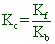Kc is called as equilibrium constant expressed in terms of molar concentrations. It is the ratio of products of equilibrium concentrations of products to the product of equilibrium concentrations of reactants. In general, it can be represented as: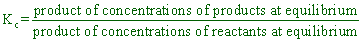Units of Kc: Most of the times the Kc is expressed with out units. However the units of Kc can be given as (mol.L-1)Δn

Where Δn = (c+d) - (a+b) = (total no. of moles of products) - (total no. of moles of reactants).

Note: These no. of moles are the stoichiometric coefficients in the balanced chemical equation for the equilibrium. While counting this number, the no. of moles of solids and liquids are not taken into consideration.

Factors affecting the equilibrium constant:

* There is no effect of pressure, concentration and catalyst on the value of equilibrium constant.

* However, the equilibrium constant depends on the temperature.

Usually, in exothermic reactions,  increase in temperature decreases the the equilibrium constant, Kc

Whereas, in endothermic reactions, increase in temperature increases the Kc value.

## EQUILIBRIUM CONSTANT, Kp (IN TERMS OF PARTIAL PRESSURES)

Kp is the equilibrium constant in terms of partial pressures. Let A, B, C and D are gases in the following reaction.Then for the above reaction, the Kp can be written as: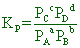Where PA, PB, PC and PD are the partial pressures of A, B, C and D at equilibrium.

## RELATION BETWEEN Kc & Kp

Derivation:

From the ideal gas equation;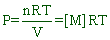Where [M] = molar concentration.

Now we can write: PA = [A]RT, PB = [B]RT, PC = [C]RT & PD = [D]RT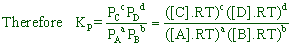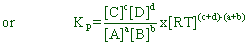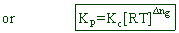Where Δng = (no. of moles of gaseous products) - (no. of moles of gaseous reactants).

Note: These no. of moles are the stoichiometric coefficients of only gaseous reactants and products in the balanced chemical equation for the equilibrium.

The following conclusions can be drawn from above equation:

If Δng = 0 then Kp = Kc

If Δng > 0 then Kp > Kc

If Δng < 0 then Kp < Kc

## ILLUSTRATIONS

The expressions for Kc and Kp and the relation between them for some reversible reactions are illustrated below.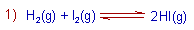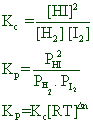Since Δng = (2) - (1+1) = 0

Kp = Kc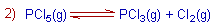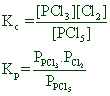Since Δng = (1+1) - (1) = 1

Kp > Kc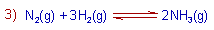Since Δng = (2) - (1+3) = -2

Kp < Kc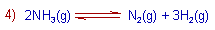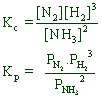Since Δng = (1+3) - (2) = 2

Kp > Kc

i.e., The expressions for Kc and Kp and the relation between them depends on how we expressed the reversible reaction as the stoichiometric equation.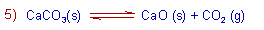KC = [CO2]Since Δng = (1) - (0) = 1

Kp > Kc

In above expressions, the active masses of solids are taken as one and hence do not appear in the expressions.

## REACTION QUOTIENTS, Q & QP

The reaction quotient, Q is defined as the ratio of products of concentrations of products to the product of concentrations of reactants at an instant of time.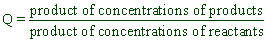i.e., The equilibrium constant, Kc is the special case of reaction quotient, Q.

If the Q value is equal to Kc, the reaction is said to be at equilibrium.

If the Q value is not equal to Kc, the reaction has to be proceeded either to the right (towards the products side) or to the left (towards the reactants side) to reach the equilibrium state.

For example, if Q < KC, the reactants concentration has to be decreased to make Q equals to KC. Hence the forward reaction is favored to restore the equilibrium.

Else if, Q > KC, then the products concentration must be decreased to make Q equals to KC again. Hence the backward reaction is favored to restore the equilibrium.

In the same way, the reaction quotient in terms of partial pressures, Qp can be defined. It is the ratio of product of partial pressures of products to product of partial pressures of reactants at any instant of time.

The same arguments about Q and Kc are valid for Qp and Kp.